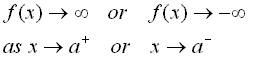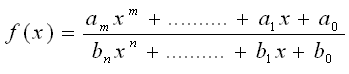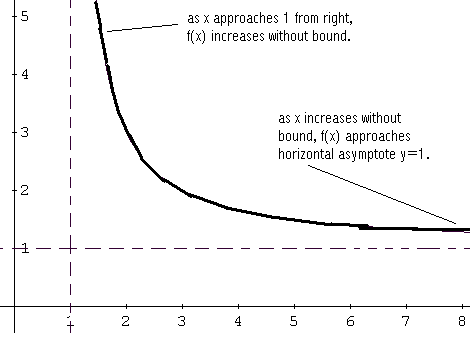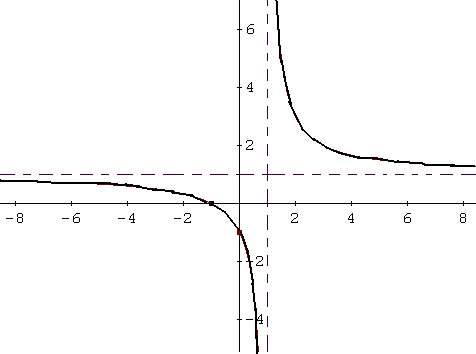# Graphing Rational Functions

How to graph a rational function? A step by step tutorial. The properties such as domain, vertical and horizontal asymptotes of a rational function are also investigated. Free graph paper is available.

## Definition of a Rational Function

A rational function f has the form $f(x) = \dfrac{g(x)}{h(x)}$ where $g (x)$ and $h (x)$ are polynomial functions.
The domain of $f$ is the set of all real numbers except the values of $x$ that make the denominator $h (x)$ equal to zero.
In what follows, we assume that $g (x)$ and $h (x)$ have no common factors.

## Vertical Asymptotes of Rational Functions

Let $f(x) = \dfrac{2}{x-3}$
The domain of $f$ is the set of all real numbers except 3, since 3 makes the denominator equal to zero and the division by zero is not allowed in mathematics. However we can try to find out how does the graph of $f$ behave close to 3.
let us evaluate function $f$ at values of $x$ close to 3 such that $x \lt 3$. The values are shown in the table below:

 $x$ 1 2 2.5 2.8 2.9 2.99 2.999 2.99999 $f(x)$ -1 -2 -4 -10 -20 -200 -2000 -2 �105

Let us now evaluate $f$ at values of $x$ close to 3 such that $x \gt 3$.

 $x$ 5 4 3.5 3.2 3.1 3.01 3.001 3.00001 $f(x)$ 1 2 4 10 20 200 2000 2�105

The graph of $f$ is shown below.Notes that
1) As $x$ approaches 3 from the left or by values smaller than 3, $f (x)$ decreases without bound.
2) As $x$ approaches 3 from the right or by values larger than 3, $f (x)$ increases without bound. We say that the line $x = 3$, broken line, is the vertical asymptote for the graph of $f$. In general, the line $x = a$ is a vertical asymptote for the graph of f if $f (x)$ either increases or decreases without bound as x approaches a from the right or from the left. This is symbolically written as:## Horizontal Asymptotes of Rational Functions

Let $f(x) = \dfrac{2x + 1}{x}$ 1) Let $x$ increase and find values of $f (x)$.

 $x$ 1 10 103 106 f (x) 3 2.1 2.001 2

2) Let $x$ decrease and find values of $f (x)$.

 $x$ -1 -10 -103 -106 $f(x)$ 1 1.9 1.999 2

As $| x |$ increases, the numerator is dominated by the term $2 x$ and the numerator has only one term x. Therefore $f(x)$ takes values close to $\dfrac{2x}{2} = 2$. See graphical behaviour below.In general, the line $y = b$ is a horizontal asymptote for the graph of $f$ if $f (x)$ approaches a constant $b$ as $x$ increases or decreases without bound.
How to find the horizontal asymptote?
Let $f$ be a rational function defined as followsTheorem
$m$ is the degree of the polynomial in the numerator and $n$ is the degree of the polynomial in the numerator.
case 1: For $m \lt n$ , the horizontal asymptote is the line $y = 0$.
case 2: For $m = n$ , the horizontal asymptote is the line $y = a_m / b_n$

case 3: For $m \gt n$ , the graph has no horizontal asymptote.

### Example 1

Let $f$ be a rational function defined by
$f(x) = \dfrac{x + 1}{x-1}$
a - Find the domain of $f$.
b - Find the $x$ and $y$ intercepts of the graph of $f$.
c - Find the vertical and horizontal asymptotes for the graph of $f$ if there are any.
d - Use your answers to parts a, b and c above to sketch the graph of function $f$.

Detailed Solution to Example 1
a - The domain of $f$ is the set of all real numbers except $x = 1$ , since this value of $x$ makes the denominator zero.
b - The $x$ intercept is found by solving $f (x) = 0$ or $x+1 = 0$. The x intercept is at the point $(-1 , 0)$.
The $y$ intercept is at the point $(0 , f(0)) = (0 , -1)$.
c - The vertical asymptote is given by the zero of the denominator $x = 1$.
The degree of the numerator is 1 and the degree of the denominator is 1. They are equal and according to the theorem above, the horizontal asymptote is the line $y = 1 / 1 = 1$.
d - Although parts a, b and c give some important information about the graph of $f$, we still need to construct a sign table for function f in order to be able to sketch with ease.
The sign of $f (x)$ changes at the zeros of the numerator and denominator. To find the sign table, we proceed as in solving rational inequalities. The zeros of the numerator and denominator which are -1 and 1 divides the real number line into 3 intervals:
$(- \infty , -1) , (-1 , 1) , (1 , + \infty)$
We select a test value within each interval and find the sign of $f (x)$.
In the interval $(- \infty , -1)$ , select -2 and find $f (-2) = ( -2 + 1) / (-2 - 1) = 1 / 3 \gt 0$.
In $(-1 , 1)$ , select 0 and find $f(0) = -1 \lt 0$.
In $(1 , + \infty)$ , select 2 and find $f (2) = ( 2 + 1) / (2 - 1) = 3 \gt 0$.

Let us put all the information about function $f$ in a table.

 x - ∞ -1 1 + ∞ f (x) + 0 x-intercepts - V.A. +
In the table above V.A means vertical asymptote.
To sketch the graph of $f$, we start by sketching the $x$ and $y$ intercepts and the vertical and horizontal asymptotes in broken lines. See sketch below.We now start sketching the graph of f starting from the left.
In the interval $(-\infty , -1)$ , $f (x)$ is positive hence the graph is above the $x$ axis. Starting from left, we graph $f$ taking into account the fact that $y = 1$ is a horizontal asymptote: The graph of $f$ is close to this line on the left. See graph below.Between -1 and 1 $f (x)$ is negative, hence the graph of $f$ is below the x axis. $(0 , -1)$ is a y intercept and $x =1$ is a vertical asymptote: as x approaches 1 from left $f (x)$ deceases without bound because $f (x) \lt 0$ in $( -1 , 1)$. See graph below.For $x \gt 1$ , $f (x) \gt 0$ hence the graph is above the x axis. As x approaches 1 from the right, the graph of f increases without bound ( $f(x) \gt 0$ ). Also as x increases, the graph of f approaches $y = 1$ the horizontal asymptote. See graph below.We now put all "pieces" of the graph of f together to obtain the graph of f.### Example 2

Let $f$ be a rational function defined by
$f(x) = \dfrac{-x + 2}{x+4}$
a - Find the domain of $f$.
b - Find the x and y intercepts of the graph of $f$.
c - Find the vertical and horizontal asymptotes for the graph of $f$ if there are any.
d - Use your answers to parts a, b and c above to sketch the graph of function $f$.

a) All real numbers except $x = - 4$
b) x intercept at $(2 , 0)$ , y intercept at $(0 , 1/2)$.
c) vertical asymptote: $x = - 4$ , horizontal asymptote: $y = - 1$.
d) The graph is shown below.## More References and Links on Graphing and Rational Functions

Graphing Functions
Rational Functions - Applet
Solver to Analyze and Graph a Rational Function